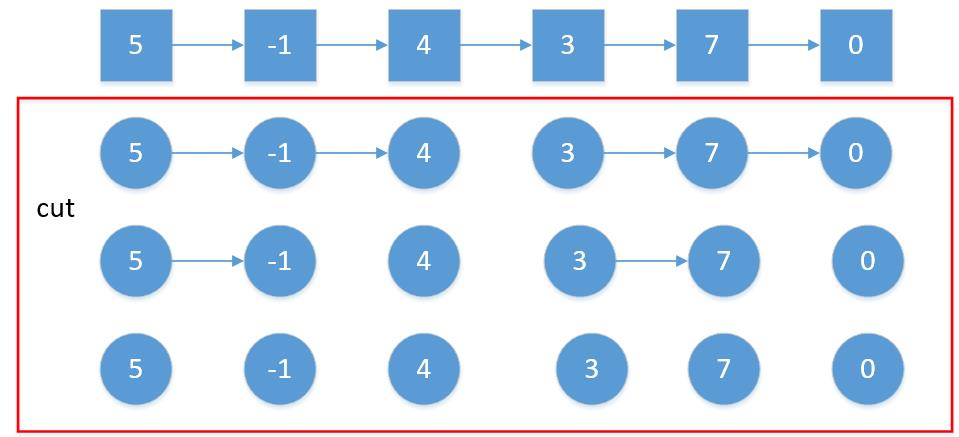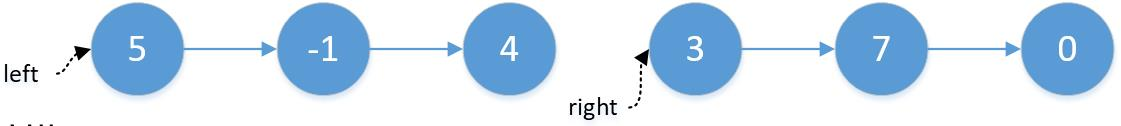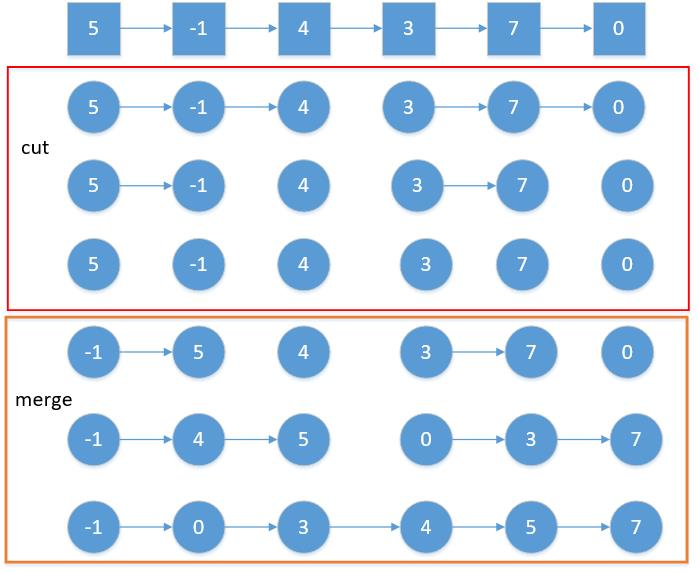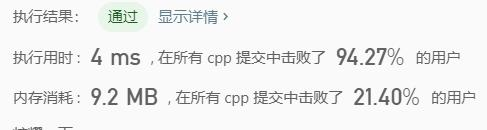# Leetcode 148：排序链表

## 解题思路## 代码实现

class Solution {public: ListNode* cut(ListNode* head, int n){ auto p = head; while (--n && p) { p = p->next; } if (!p) return nullptr; auto next = p->next; p->next = nullptr; return next;}//合并两个有序链表的子程序即可ListNode* merge(ListNode* l1, ListNode* l2){ ListNode dummy(0); auto p = &dummy; while (l1 && l2) { if (l1->val < l2->val) { p->next = l1; p = l1;//或者写p=p->next也可以 l1 = l1->next; } else { p->next = l2; p = l2;//或者写p=p->next也可以 l2 = l2->next; } } p->next = (l1 ? l1 : l2); return dummy.next;}ListNode* sortList(ListNode* head) { ListNode dummy(0); dummy.next = head;//dummyHead auto p = head; int length = 0; while (p)// the length of this linkedlist { ++length; p=p->next; } for (int size = 1; size < length; size <<= 1) { auto cur = dummy.next; auto tail = &dummy; while (cur) { auto left = cur; auto right = cut(left, size);//left->@->@ |断开|right->@->@->@... cur = cut(right, size);//left->@->@|断开|right->@->@ |断开|cur->@->... tail->next = merge(left, right); while (tail->next) { tail = tail->next; } } } return dummy.next;}};

## 复杂度# Leetcode 152：乘积最大子序列

## 解题思路

imax = max(imax * nums[i], nums[i]);

 imin = min(imin * nums[i], nums[i]);

## 代码实现

int maxProduct(vector<int>& nums) {    int maxvalue = INT_MIN, imax = 1, imin = 1;    for (int i = 0; i < nums.size(); i++)    {        if (nums[i] < 0)        {            int tmp = imax;            imax = imin;            imin = tmp;        }        imax = max(imax * nums[i], nums[i]);        imin = min(imin * nums[i], nums[i]);        maxvalue = max(maxvalue, imax);    }    return maxvalue;}

## 复杂度